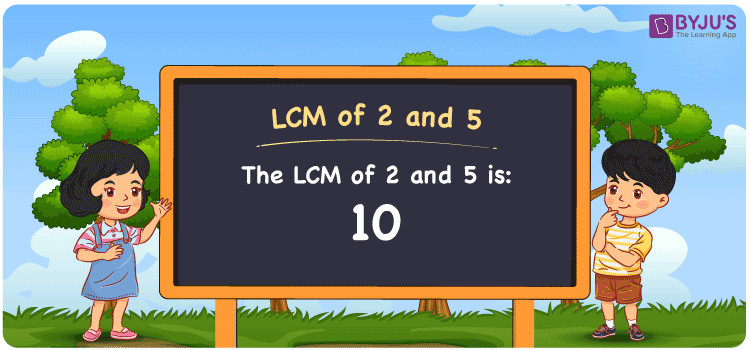Checkout JEE MAINS 2022 Question Paper Analysis : Checkout JEE MAINS 2022 Question Paper Analysis :

# LCM of 2 and 5

LCM of 2 and 5 is 10. The LCM is the method used to find the smallest common multiple between the two given numbers. Least common multiples of 2 and 5 can be found from the common multiples of the numbers. (2, 4, 6, 8, 10, 12, 14, 16, ….) and (5, 10, 15, 20, 25, 30, 35, ….) are the multiples of 2 and 5. The LCM of two numbers is found using methods like listing the multiples, prime factorization and division method.

## What is LCM of 2 and 5?

The answer to this question is 10. The LCM of 2 and 5 using various methods is shown in this article for your reference. The LCM of two non-zero integers, 2 and 5, is the smallest positive integer 10 which is divisible by both 2 and 5 with no remainder.## How to Find LCM of 2 and 5?

LCM of 2 and 5 can be found using three methods:

• Prime Factorisation
• Division method
• Listing the multiples

### LCM of 2 and 5 Using Prime Factorisation Method

The prime factorisation of 2 and 5, respectively, is given by:

2 = 2¹

5 = 5¹

LCM (2, 5) = 10

### LCM of 2 and 5 Using Division Method

We’ll divide the numbers (2, 5) by their prime factors to get the LCM of 2 and 5 using the division method (preferably common). The LCM of 2 and 5 is calculated by multiplying these divisors.

 2 2 5 5 1 5 1 1

No further division can be done.

Hence, LCM (2, 5) = 10

### LCM of 2 and 5 Using Listing the Multiples

To calculate the LCM of 2 and 5 by listing out the common multiples, list the multiples as shown below

 Multiples of 2 Multiples of 5 2 5 4 10 6 15 8 20 10 25

LCM (2, 5) = 10

## Video Lesson on Applications of LCM## LCM of 2 and 5 Solved Examples

Determine the smallest number which is exactly divisible by 2 and 5.

Solution:

We know that

LCM is the smallest number exactly divisible by 2 and 5.

Multiples of 2 = 2, 4, 6, 8, 10, 12, 14, 16, ….

Multiples of 5 = 5, 10, 15, 20, 25, 30, 35, ….

Hence, the LCM of 2 and 5 is 10.

## Frequently Asked Questions on LCM of 2 and 5

### List the methods used to find the LCM of 2 and 5.

The methods used to find the LCM of 2 and 5 are Prime Factorisation, Division method and Listing the multiples

### By using the prime factorisation method, determine the LCM of 2 and 5.

To find the LCM, the factors must be known

2 = 2¹

5 = 5¹

LCM is the product of prime factors raised to the highest exponent among 2 and 5.

LCM of 2 and 5 = 2¹ x 5¹ = 10

### What is the GCF if the LCM of 2 and 5 is 10?

LCM x GCF = 2 x 5

As the LCM = 10

10 x GCF = 10

GCF = 10/10 = 1